## Tamilnadu Samacheer Kalvi 10th Maths Solutions Chapter 4 Geometry Ex 4.4

10th Maths Exercise 4.4 Samacheer Kalvi Question 1.
The length of the tangent to a circle from a point P, which is 25 cm away from the centre is 24 cm. What is the radius of the circle?
Solution:
242 + r2 = 252
576 + r2 = 625
r2 = 625 – 576
= 49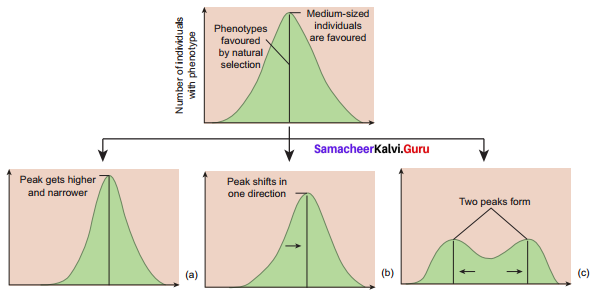Ex 4.4 Class 10 Samacheer Question 2.
∆LMN is a right angled triangle with ∠L = 90°. A circle is inscribed in it. The lengths of the sides containing the right angle are 6 cm and 8 cm. Find the radius of the circle.
Solution:
∆LMN,
By Pythagoras theorem,Exercise 4.4 Class 10 Samacheer Kalvi Question 3.
A circle is inscribed in ∆ABC having sides 8 cm, 10 cm and 12 cm as shown in figure, Find AD, BE and CF.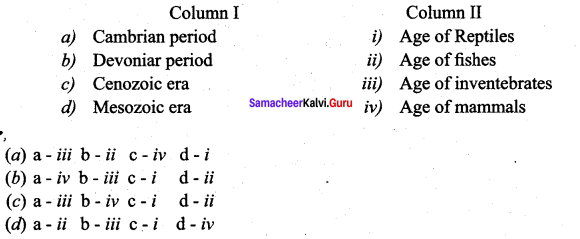Solution:
We know that the tangents drawn from are external point to a circle are equal.
Therefore AD = AF = x say.
BD = BE = y say and
CE = CF = z say
Now, AB = 12 cm, BC = 8 cm, and CA= 10 cm.
x + y = 12, y + z = 8 and z + x = 10
(x + y) + (y + z) + (z + x) = 12 + 8 + 10
2(x + y + z) = 30
x + y + z = 15
Now, x + y = 12 and x + y + z = 15
12 + z = 15 ⇒ z = 3
y + z = 8 and x + y + z = 15
x + 8 = 15 ⇒ x = 7
and z + x = 10 and x + y + z = 15
10 + y = 15 ⇒ y = 5
Hence, AD = x = 7 cm, BE = y = 5 cm
and CF = z = 3 cm.

10th Maths Exercise 4.4 Question 4.
PQ is a tangent drawn from a point P to a circle with centre O and QOR is a diameter of the circle such that ∠POR = 120° . Find ∠OPQ.
Solution:
∠POR + ∠POQ = 180° (straight angle = 180°)
∴ 120 +∠POQ = 180°
∠POQ = 60°
∠OQP = 90° (∵ radius is ⊥r to the tangent at the point of contact)∴ ∠POQ + ∠PQO + ∠OPQ = 180° (∵ sum of the 3 angles of a triangle is 180°)
∴ 60 + 90 + ∠OPQ = 80°
∠OPQ = 180° – 150° = 30°

10th Maths Geometry Question 5.
A tangent ST to a circle touches it at B. AB is a chord such that ∠ABT = 65°. Find ∠AOB , where “O” is the centre of the circle.
Solution:
In the figure,
∠OBT = 90° (∵OB-radius, BT – Tangent)
= 115°
∴ ∠OBA = 90° – 65°
∠OAB = 25° (OA = OB)
∴ ∠AOB = 180° – 50°
= 130°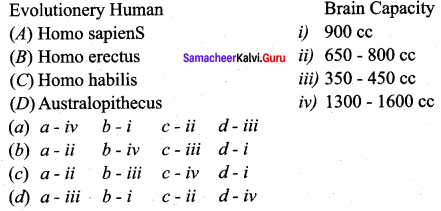10th Maths Practical Geometry Guide Pdf Question 6.
In figure, O is the centre of the circle with radius 5 cm. T is a point such that OT = 13 cm and OT intersects the circle E, if AB is the tangent to the circle at E, find the length of AB.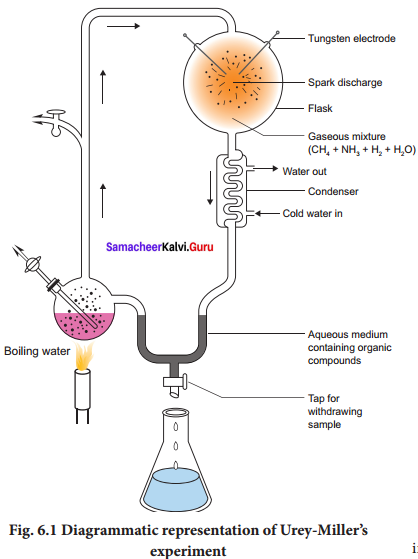Solution:
In ∆OPT, OP = r = 5 cm
OT = 13 cm
PT = 12 cm
In ∆OPA, OA2 = OP2 + AP2 ………….. (1)
In ∆OAE, OA2 = OE2 + AE2 …………. (2)
Equating (1) and (2),
OP2 + AP2 = OE2 + AE2 (∵ OP = OE = r)
∴ AP = AE
Parallel BQ = EB
In ∆AET, AT2 = AE2 + ET2
∴ ET2 = AT2 – AE2 = (AT + AE) (AT – AE)
∴ ET2 = (AT + AP) (AT – AE) (∵ AE = AP)
∴ 8 × 8 = 12 × (AT – AE)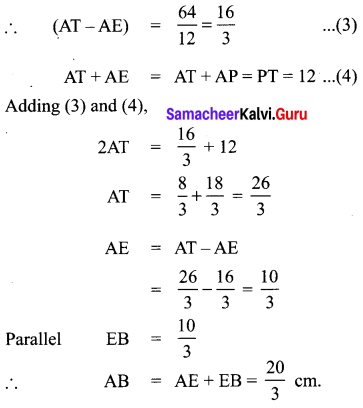10th Geometry Question 7.
In two concentric circles, a chord of length 16 cm of larger circle becomes a tangent to the smaller circle whose radius is 6 cm. Find the radius of the larger circle.
Solution:
AB = 16 cm given
CA = CB(∵ OC ⊥r AB)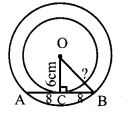OB2 = OC2 + BC2
= 62 + 82
= 36 + 64 = 100
OB = Radius of the larger circle = $$\sqrt{100}$$ = 10 cm.

10th Geometry Exercise 4.4 Question 8.
Two circles with centres O and O’ of radii 3 cm and 4 cm respectively intersect at two points P and Q, such that OP and O’P are tangents to the two circles. Find the length of the common chord PQ.
Solution:
Given: OP = OQ = 4
O’P = O’Q = 3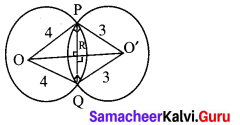OO’ is the perpendicular bisector of chord PQ.
Let R be the point of intersection of PQ and OO’.
Assume PR = QR = x and OR = y
In OPO’, OP2 + O’P2 = (OO’)2 ⇒ OO’
= $$\sqrt{4^{2}+3^{2}}$$ = 5
OR = y ⇒ OR = 5 – y
In ∆OPR, PR2 + OR2 = OP2 ⇒ x2 + y = 42 ……….. (1)
In ∆O’PR, PR2 + O’R2 = O’P2 ⇒ x2 + (5 – y)2 = 9 ………….(2)
(1) – (2)=> y2 – (25 + y2 – 10y) = 16 – 9
⇒ y2 – 25 – y2 + 10y = 7 .
⇒ 10y = 25 + 7 ⇒ 10y =32
⇒ y =3.2
Substituting y = 3.2 in (1), we get x = $$\sqrt{4^{2}-3.2^{2}}$$
x = 2.4
PQ = 2x ⇒ PQ = 4.8 cm

10th Maths Geometry Exercise 4.4 Question 9.
Show that the angle bisectors of a triangle are concurrent.
Solution: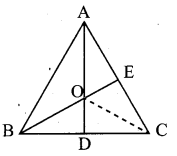In ∆ABC, let AD, BE are two angle bisectors.
They meet at the point ‘O’
We have to prove that = $$\frac{A C}{C D}=\frac{A O}{O D}$$
Construct CO to meet the interesecting point O from C.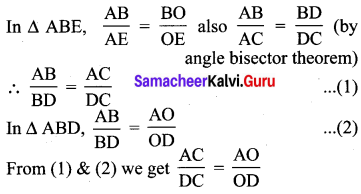Hence proved.

Samacheer Kalvi 10th Maths Practical Geometry Question 10.
In ∆ABC , with ∠B = 90° , BC = 6 cm and AB = 8 cm, D is a point on AC such that AD = 2 cm and E is the midpoint of AB. Join D to E and extend it to meet at F. Find BF.
In the figure ∆ABC, ∆EBF are similar triangles.
Solution:
Consider DABC, Then D, E, F are respective points on the sides CA, AB and BC. By constrution D, E, F are collinear.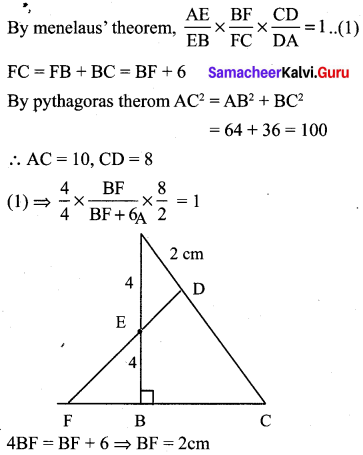10th Maths Guide Geometry Question 11.
An artist has created a triangular stained glass window and has one strip of small length left before completing the window. She needs to figure out the length of left out portion based on the lengths of the other sides as shown in the figure.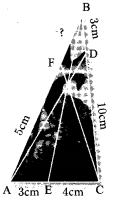Solution:
In the figure, let O be the concurrent point of the angle bisectors of the three angles.10th Maths Practical Geometry Question 12.
Draw a tangent at any point R on the circle of radius 3.4 cm and centre at P ?
Solution:
Centre = P
Tangent at any point R.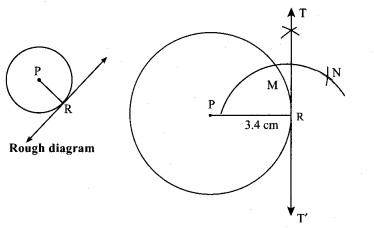Construction:
Steps:
(1) Draw a circle with centre P of radius 3.4 cm.
(2) Take a point R on the circle. Join PR.
(3) Draw ⊥r line TT1 to PR. Which passes through R.
(4) TT1 is the required tangent.

10th Maths Geometry Guide Question 13.
Draw a circle of radius 4.5 cm. Take a point on the circle. Draw the tangent at that point using the alternate segment theorem.
Solution:
Construction:
Steps:
(1) With O as the centre, draw a circle of radius 4.5 cm.
(2) Take a point R on the circle. Through R draw any chord PR.
(3) Take a point Q distinct from P and R on the circle, so that P, Q, R are in anti-clockwise direction. Join PQ and QR.
(4) Through R drawn a tangent TT1 such that ∠TRP = ∠PQR.
(5) TT1 is the required tangent.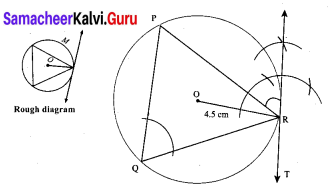Maths Geometry 10th Question 14.
Draw the two tangents from a point which is 10 cm away from the centre of a circle of radius 5 cm. Also, measure the lengths of the tangents.
Solution:
The distance between the point from the centre is 10 cm.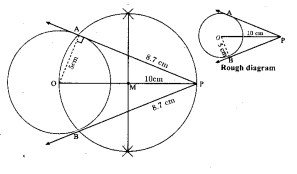Construction:
Steps:
(1) With O as centre, draw a circle of radius 5 cm.
(2) Draw a line OP =10 cm.
(3) Draw a perpendicular bisector of OP which cuts OP at M.
(4) With M as centre and MO as radius, draw a circle which cuts previous circle at A and B.
(5) Join AP and BP. AP and BP are the required tangents. Thus length of the tangents are PA and PB = 8.7 cm.
Verification:
In the right triangle ∠POA;10th Maths New Syllabus Exercise 4.4 Question 15.
Take a point which is 11 cm away from the centre of a circle of radius 4 cm and draw the two tangents to the circle from that point.
Solution:
The distance of a point from the center =11 cm.Construction:
Steps:
(1) With centre O, draw a circle of radius 4 cm.
(2) Draw a line OP = 11 cm.
(3) Draw a ⊥r bisector of OP, which cuts atM.
(4) With M as centre and MO as radius, draw a circle which cuts previous circle at A and B.
(5) Join AP and BP. AP and BP are the required tangents. Thus length of the tangents are PA = PB = 10.2 cm.
Verification:
In the right triangle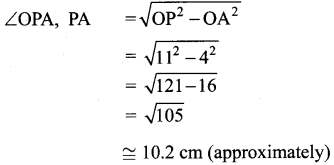10th Maths 4.4 Question 16.
Draw the two tangents from a point which is 5 cm away from the centre of a circle of diameter 6 cm. Also, measure the lengths of the tangents.
Solution:
Diameter = 6 cm
Radius = $$\frac{6}{2}$$ = 3 cm.
The distance between the centre and the point is 5 cm.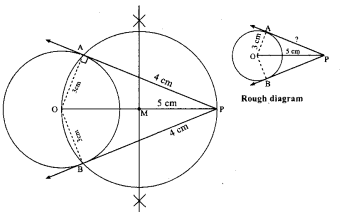Construction:
Steps:
(1) With centre O, draw a circle of radius , 3cm.
(2) Draw a line OP = 5 cm.
(3) Draw a bisector of OP, which cuts OP and M.
(4) With M as centre and MO as radius draw a circle which cuts previous circle at A and B.
(5) Join AP and BP. AP and BP are the required tangents. Thus length of the tangents are PA = PB = 4 cm
Verification:
In the right triangle ∆OPA,10th Maths Book Geometry Question 17.
Draw a tangent to the circle from the point P having radius 3.6 cm, and centre at O. Point P is at a distance 7.2 cm from the centre.